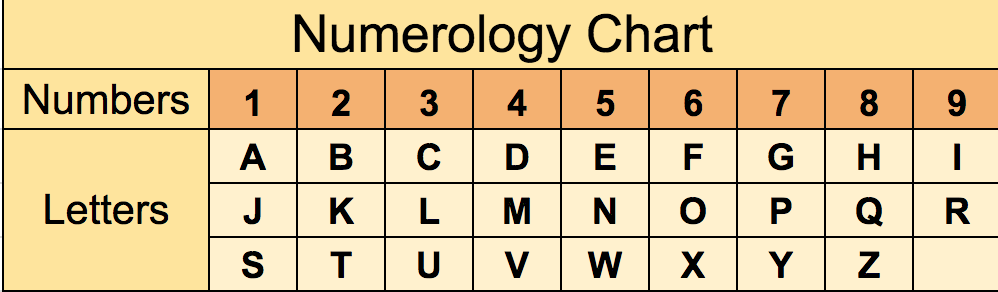Numerology chart provides number equivalents of letters in the alphabet.

To calculate numbers in numerology, the numerology chart (table) is frequently used. As you probably know, in numerology, all letters in the first and last name are converted to numbers. These conversions are made based on the numerology chart.

In the numerology calculator below, we use pythagorean numerology chart. There is also chaldean numerology chart that we don't utilize. The chart has the letters in English alphabet.

For other alphabets like Turkish or Spanish, there are different letters like ş or ñ. In this case, that letter is converted to the most similiar letter in the English alphabet. Like ş is considered as s.

Enter your full name (given at birth with surname) and date of birth to the numerology calculator below then click on the calculate button to see your numerology numbers with free numerology report and numerology reading.

There is also an exception for calculating numerology numbers based on only vowels or consonants. In this case, y can be counted as both a vowel and a consonant. If letter y sounds like a vowel when reading the name, then consider it as i. Otherwise, consider it as y.

## Pythagorean Numerology ChartYou can comment on numerology calculator or ask questions below: